# AP Board 9th Class Maths Solutions Chapter 2 Polynomials and Factorisation Ex 2.4

AP State Syllabus AP Board 9th Class Maths Solutions Chapter 2 Polynomials and Factorisation Ex 2.4 Textbook Questions and Answers.

## AP State Syllabus 9th Class Maths Solutions 2nd Lesson Polynomials and Factorisation Exercise 2.4

Question 1.
Determine which of the following polynomials has (x + 1) as a factor.
i) x3 – x2 – x + 1
Solution:
f(- 1) = (- 1)3 – (- 1)2 – (- 1) + 1
= -1 – 1 + 1 + 1 = 0
∴ (x + 1) is a factor.ii) x4 -x3 +x2 – x + 1
Solution:
f(- 1) = (- 1)4 – (- 1)3 + (- 1)2 – (- 1) + 1
= 1 + 1 + 1 + 1 + 1= 5
∴ (x + 1) is not a factor.

iii) x4 + 2x3 + 2x2 + x + 1
Solution:
f(- 1) = (-1)4 + 2 (- 1)3 + 2 (- 1)2 + (-1) + 1
= 1 – 2 + 2 – 1 + 1 = 1
∴ (x + 1) is not a factor.

iv) x3 – x2 – (3 – √3)x + √3
Solution:
f(- 1) = (- 1)3 – (- 1)2 – (3 – √3)(-1) + √3
= – 1 – 1 + 3 – √3 + √3 = 1
∴ (x + 1) is not a factor.

Question 2.
Use the factor theorem to determine whether g(x) is a factor of f(x) in each of the following cases:
i) f(x) = 5x3 + x2 – 5x – 1; g(x) = x + 1
[Factor theorem : If f(x) is a polynomial; f(a) = 0 then (x – a) is a factor of f(x); a ∈ R]
Solution:
g(x) = x+ 1 = x- a say
∴ a = – 1
f(a) = f(- 1) = 5 (- 1)3 + (- 1)2 – 5 (- 1) – 1
= -5 + 1 + 5 – 1 = 0
∴ x + 1 is a factor of f(x).ii) f(x) = x3 + 3x2 + 3x + 1; g(x) = x + 1
Solution:
g(x) = x + 1 = x – a
∴ a = – 1
f(a) = f(- 1) = (- 1)3 + 3 (- 1)2 + 3(-1) + 1
= -1 + 3 – 3 + 1 =0
∴ f(x) is a factor of g(x).

iii) f(x) = x3 – 4x2 + x + 6;
g(x) = x – 2
Solution:
g(x) = x- 2 = x- a
∴ a = 2
f(a) = f(2) = 23 – 4(2)2 + 2 + 6
= 8 – 16 + 2 + 6 = 0
∴ g(x) is a factor of f(x).iv) f(x) = 3x3+ x2 – 20x +12; g(x) = 3x – 2
Solution:
g(x) = 3x – 2 = $$x-\frac{2}{3}$$ = x – a
∴ a = 2/3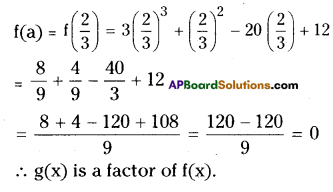v) f(x) = 4x3+ 20x2+ 33x + 18; g(x) = 2x + 3
Solution:
g(x) = 2x + 3 = x + $$\frac{3}{2}=$$ = x – a
∴ a = -3/2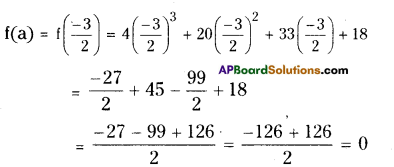∴ g(x) is a factor of f(x).Question 3.
Show that (x – 2), (x + 3) and (x – 4) are factors of x3 – 3x2 – 10x + 24.
Solution:
Given f(x) = x3 – 3x2 – 10x + 24
To check whether (x – 2), (x + 3) and (x – 4) are factors of f(x), let f(2), f(- 3) and f(4)
f(2) = 23 – 3(2)2 – 10(2) + 24
= 8- 12-20 + 24 = 0
∴ (x – 2) is a factor of f(x).

f(- 3) = (- 3)3 – 3(- 3)2– 10(- 3) + 24
= – 27 – 27 + 30 + 24 = 0
∴ (x + 3) is a factor of f(x).

f(4) = (4)3 – 3 (4)2 – 10 (4) + 24
= 64 – 48 – 40 + 24
= 88 – 88
= 0
∴ (x – 4) is a factor of f(x).

Question 4.
Show that (x + 4), (x – 3) and (x – 7) are factors of x3 – 6x2 – 19x + 84.
Solution:
Let f(x) = x3 – 6x2 – 19x + 84
To verify whether (x + 4), (x – 3) and (x – 7) are factors of f(x) we use factor theorem.

Let f(- 4), f(3) and f(7)
f(- 4) = (- 4)3 – 6 (- 4)2 – 19 (- 4) + 84
= -64 – 96 + 76 + 84
= 0 .
∴ (x + 4) is a factor of f(x).

f(3) = 33 – 6(3)2 – 19(3) + 84
= 27 – 54 – 57 + 84
= 0
∴ (x – 3) is a factor of f(x).f(7) = 73 – 6(7)2 – 19(7) + 84
= 343 – 294 – 133 + 84
= 427 – 427
= 0
∴ (x – 7) is a factor of f(x).

Question 5.
If both (x – 2) and $$\left(x-\frac{1}{2}\right)$$ of px2 + 5x + r, show that p = r.
Solution:
Let f(x) = px2+ 5x + r
As (x – 2) and $$\left(x-\frac{1}{2}\right)$$ are factor of f(x), we have f(2) = 0 and f(1/2) = 0
∴ f(2) = p(2)2 + 5(2) + r
= 4p + 10 + r = 0
= 4p + r
= – 10 ………………(1)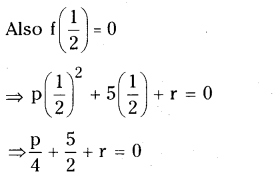⇒ p + 10 + 4r = 0
⇒ p + 4r = – 10 ………………. (2)
From (1) and (2);
4p + r = p + 4r
4p – p = 4r – r
3p = 3r
∴ P = rQuestion 6.
If (x2 – 1) is a factor of ax4 + bx3 + cx2 + dx + e, show that a + c + e = b + d = 0.
Solution:
Let f(x) = ax4 + bx3 + cx2 + dx + e
As (x – 1) is a factor of f(x) we have
x2 – 1 = (x + 1) (x – 1) hence f(1) = 0 and f(-1) = 0
f(1) = a + b + c + d + e = 0 ……………. (1)
and f(-1) = a- b + c- d + e = 0
⇒ a + c + e = b + d
Substitute this value in equation (1)
a + c + e + b + d=0
b + d + b + d=0
2 (b + d) = 0
⇒ b + d = 0
∴ a + c + e = b + d = 0Question 7.
Factorise
i) x3 – 2x2 – x + 2
Solution:
Let f(x) = x3 – 2x2 – x + 2
By trial, we find f(l) = 13 – 2(1)2 – 1 + 2
= 1 – 2 – 1 + 2
= 0 .
∴ (x – 1) is a factor of f(x).
[by factor theorem]
Now dividing f(x) by (x – 1).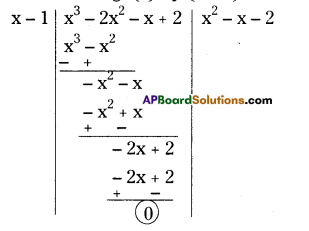f(x) = (x – 1) (x2 – x – 2)
= (x – 1) [x2 – 2x + x- 2]
= (x – 1) [x (x – 2) + 1 (x – 2)]
= (x – 1) (x – 2) (x + 1)

ii) x3 – 3x2 – 9x – 5
Solution:
Let f(x) = x3 – 3x2 – 9x – 5By trial,
f(- 1) = (- 1)3 – 3(- 1)2 – 9(- 1) – 5
=-1 – 3 + 9 – 5
=0
∴ (x + 1) is a factor of f(x).
[ ∵ by factor theorem]
Now dividing f(x) by (x + 1).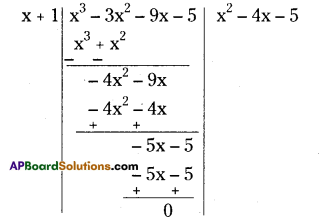f(x)=(x + 1)(x2 – 4x – 5)
But x2– 4x – 5 = x2 – 5x + x – 5
= x (x – 5) + 1 (x – 5)
=(x – 5)(x + 1)
∴ f(x)=(x + 1)(x + 1)(x – 5)iii) x3 + 13x2 + 32x + 20
Solution:
Let f(x) = x3 + 13x2 + 32x + 20
Let f(- 1)
= (- 1)3 + 13 (- 1)2 + 32 (- 1) + 20
= – 1 + 13 – 32 + 20 = 33 – 33 = 0
∴ (x + 1) is a factor of f(x).
[ ∵ by factor theorem] Now dividing f(x) by (x + 1).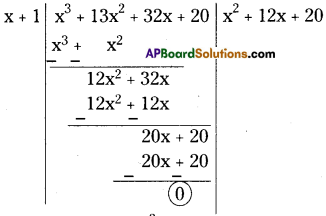iv) y3 + y2 – y – 1
Let f(y) = y3 + y2 – y – 1
f(1) = 13+ 12– 1 – 1 = 0
(y – 1) is a factor of f(y).
Now dividing f(y) by (y – 1).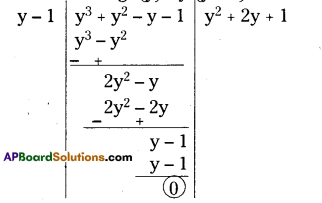∴ f(x) = (x + 1)(x2 + 12x + 20)
But (x2 + 12x + 20) = x2+ 10x + 2x + 20
=x(x + 10)+2(x + 10)
=(x + 10)(x + 2)
∴f(x) = (x + 1)(x + 2)(x + 10)Question 8.
If ax2 + bx + c and bx2 + ax + c have a common factor x + 1 then show that c = 0 and a = b.
Solution:
Let f(x) = ax2 + bx + c and g(x) = bx2 + ax + c given that (x + 1) is a common factor for both f(x) and g(x).
∴ f(-1) = g(- 1)
⇒a(- 1)2 + b(- 1) + c
= b(- 1)2 + a (- 1) + c
⇒ a – b + c = b – a + c
⇒ a + a = b + b
⇒ 2a = 2b
⇒ a = b
Also f(- 1) = a – b + c = 0
⇒ b – b + c = 0
⇒ c = 0

Question 9.
If x2 – x – 6 and x2 + 3x – 18 have a common factor x – a then find the value of a.
Solution:
Let f(x) = x2 – x – 6 and
g(x) = x2 + 3x – 18
Given that (x – a) is a factor of both f(x) and g(x).
f(a) = g(a) = 0
⇒ a2 – a – 6 = a2 + 3a – 18
⇒ – 4a = – 18 + 6
⇒ – 4a = – 12
∴ a = 3Question 10.
If (y – 3) is a factor of y3– 2y2– 9y + 18, then find the other two factors.
Solution:
Let f(y) = y3– 2y2 – 9y + 18
Given that (y – 3) is a factor of f(y).
Dividing f(y) by (y – 3)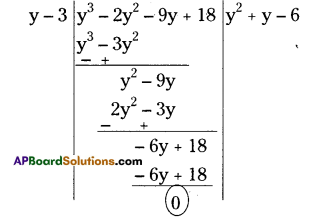∴ f(y) = (y – 3) (y + y – 6)
But y2 + y – 6
= y2 + 3y – 2y – 6
= y (y + 3) – 2 (y + 3)
= (y + 3) (y – 2)
∴ f(y) = (y – 2)(y – 3)(y + 3)
The other two factors are (y – 2) and (y + 3).